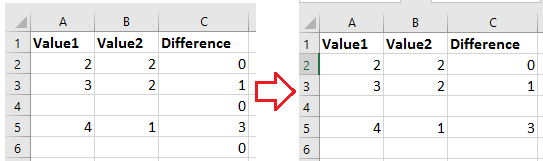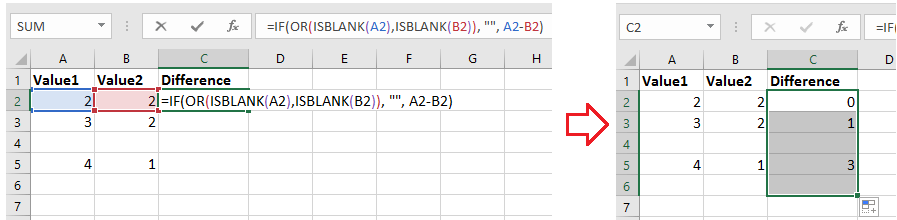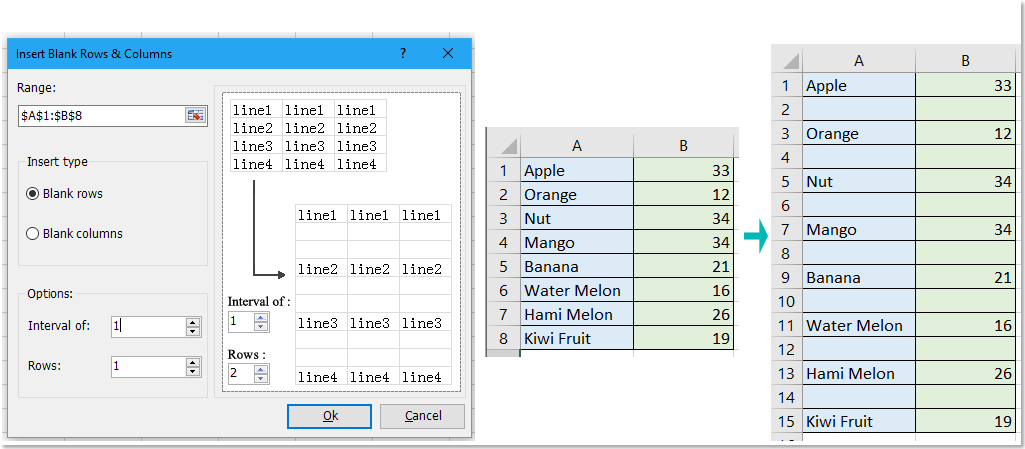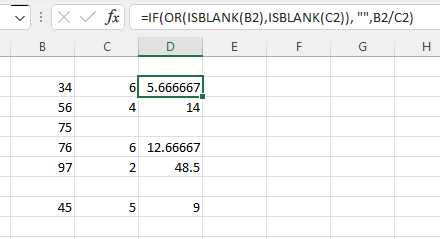## How to keep cell blank when applying formula until data entered in Excel?

In Excel, if you apply a formula to a column range, the result will be displayed as zero while the reference cells are blank in the formula. But in this case, I want to keep the cell empty when apply formula until the reference cell entered with data, if there are any tricks to handle it?Keep cell blank until data entered in

####Keep cell blank until data entered in

Actually, there is a formula that can help you to keep the formula cell empty until data entered in reference cells.

For instance, here to calculate the difference between column Value 1 and column Value 2 in column Differences, and you want to keep the cell blank if there are some blank cells in the column Value 1 and column Value2.

Select first cell that you want to place the calculated result, type this formula =IF(OR(ISBLANK(A2),ISBLANK(B2)), "", A2-B2), and drag fill handle down to apply this formula to the cells you need.In the formula, A2 and B2 are the reference cells in the formula you want to apply, A2-B2 are the calculation you want to use.

#### Batch insert blank rows or columns in a specific interval in Excel range

If you want to insert blank rows in every other row, you may need to insert them one by one, but the Insert Blank Rows & Columns of Kutools for Excel can solve this job in seconds. Click for 30 days free trial!### Best Office Productivity Tools

 Popular Features: Find, Highlight or Identify Duplicates   |  Delete Blank Rows   |  Combine Columns or Cells without Losing Data   |   Round without Formula ... Super Lookup: Multiple Criteria VLookup  |   Multiple Value VLookup  |   VLookup Across Multiple Sheets   |   Fuzzy Lookup .... Advanced Drop-down List: Quickly Create Drop Down List   |  Dependent Drop Down List   |  Multi-select Drop Down List .... Column Manager: Add a Specific Number of Columns  |  Move Columns  |  Toggle Visibility Status of Hidden Columns  |  Compare Ranges & Columns ... Featured Features: Grid Focus   |  Design View   |   Big Formula Bar   |  Workbook & Sheet Manager   |  Resource Library (Auto Text)   |  Date Picker   |  Combine Worksheets   |  Encrypt/Decrypt Cells   |  Send Emails by List   |  Super Filter   |   Special Filter (filter bold/italic/strikethrough...) ... Top 15 Toolsets:  12 Text Tools (Add Text, Remove Characters, ...)   |   50+ Chart Types (Gantt Chart, ...)   |   40+ Practical Formulas (Calculate age based on birthday, ...)   |   19 Insertion Tools (Insert QR Code, Insert Picture from Path, ...)   |   12 Conversion Tools (Numbers to Words, Currency Conversion, ...)   |   7 Merge & Split Tools (Advanced Combine Rows, Split Cells, ...)   |   ... and more

Supercharge Your Excel Skills with Kutools for Excel, and Experience Efficiency Like Never Before. Kutools for Excel Offers Over 300 Advanced Features to Boost Productivity and Save Time.  Click Here to Get The Feature You Need The Most...#### Office Tab Brings Tabbed interface to Office, and Make Your Work Much Easier

• Enable tabbed editing and reading in Word, Excel, PowerPoint, Publisher, Access, Visio and Project.
• Open and create multiple documents in new tabs of the same window, rather than in new windows.
• Increases your productivity by 50%, and reduces hundreds of mouse clicks for you every day!No ratings yet. Be the first to rate!
This comment was minimized by the moderator on the site
so at the minute i am trying to do totals on timesheets but in the formula which i have done E8-D8-0.50 in column I8 is showing as -0.50 how can i get it blank until data is inputted in those cells
This comment was minimized by the moderator on the site
Hi, Ollie, try this formula:
=IF(OR(ISBLANK(E8),ISBLANK(D8)), "",E8-D8-0.5)
This comment was minimized by the moderator on the site
Hi, Carmen,try this formula: =IF(OR(ISBLANK(B2),ISBLANK(C2)), "",B2/C2), it will keep blank until both of column C and column B are filled data.This comment was minimized by the moderator on the site
Hi, thank you much appreciated but I am still getting an error.

" There's a problem with the formula

Not trying to type a formula?
When the first character is an equal ("=") or a minus ("-") sign, Excel thinks its a formula:
you type: =1+1 the cell shows 2

To get around this try to type an apostrophe first:
'=1+1 the cell shows =1+1

I copied your code and replaced the column numbers.
This comment was minimized by the moderator on the site
Hi there, I want to create an epidemiologic formula to calculate the incidence proportion, which is the number of new cases during a specified time period / the number of people at risk. So essentially, column B divided by column C. However, I am creating the document for students of mine for their exam, so they can just type in the values and get the answer. I tried the formula you provided above =IF(OR(ISBLANK(A2),ISBLANK(B2)), "", A2-B2) but I keep getting an error. Can anyone assist?

Thanks.
This comment was minimized by the moderator on the site
Olá pessoal, parabéns pelo site, tem me ajudado bastante, segue abaixo um problema que ainda não consegui resolver, são várias situações em uma formula, segue descrição do que preciso e dados para criar o ambiente para que possa validar e quem sabe me ajudar com essa função:

Objetivo: fazer com que o Excel atualize a data de vencimento do contrato, somando a quantidade de meses da vigência, caso a renovação automática esteja "SIM" e se tiver "NÂO" ele não somará, assim um contrato que foi assinado em janeiro de 2021 e já teve sua renovação automática de 12 meses aplicada deve ter a data de vencimento atualizada para janeiro de 2023, se tivermos visualizando a planilha a partir de fevereiro de 2022, como seria essa formula?
Atualmente essa célula D1 já tem a seguinte formula que deve permanecer:
=SE(OU(ÉCÉL.VAZIA(A1);ÉCÉL.VAZIA(B1));"";(DATAM(A1;B1)))
Essa formula além de somar a data de assinatura do contrato e a vigência ela só apresenta o resultado se houver informações nas células A1 e B1, se não deixa vazia

Celular Formato Conteúdo Descrição
A1 Data Abreviada 12/11/2020 Dt Assinatura contrato
B1 Geral 12 Vigência (Meses)
C1 Geral Sim ou Não Renovação Automática
D1 Data Abreviada Formula Data Termino Contrato
This comment was minimized by the moderator on the site
Hi, Andre Oliveria, if cell A1 contains date and contract name, you need this formula
``=MID(A1,MIN(IFERROR(MIN(FIND({0,1,2,3,4,5,6,7,8,9},A1&"0123456789",1)),LEN(A1)+1)),LOOKUP(1,0*MID(A1,ROW(INDIRECT("1:"&LEN(A1))),1),ROW(INDIRECT("1:"&LEN(A1)))) + 1 - MIN(IFERROR(MIN(FIND({0,1,2,3,4,5,6,7,8,9},A1&"0123456789",1)),LEN(A1)+1)))``
to extract the date only, then use below formula
``=IF(OR(ISBLANK(B1),ISBLANK(C1)),"",IF(EDATE(B1,C1)<=NOW(),EDATE(B1,C1+1), IF(LOWER(D1)="yes", EDATE(B1,C1*2+1), EDATE(B1,C1+1))))``
to get the final date.
B1 is the extracted start date, C1 is the months, D1 is "yes"or"no".
This comment was minimized by the moderator on the site
Olá amigos, quero parabenizar a página pelas informações que me ajudaram e resolver um problema, porem agora preciso que um campo de data seja atualizada quando o campo renovação estiver como SIM, abaixo segue uma descrição e dados para ajudar, já tentei criar essa formula mais não consegui:

Celular Formato Conteúdo Descrição
A1 Data Abreviada 12/11/2020 Dt. Assinatura contrato
B1 Geral 12 Vigência (Meses)
C1 Geral Sim ou Não Renovação Automática
D1 Data Abreviada Formula Data Termino Contrato

Objetivo: fazer com que o Excel atualize a data de vencimento do contrato, somando a quantidade de meses da vigência, caso a renovação automática esteja "SIM" e se tiver "NÂO" ele não somará, assim um contrato que foi assinado em janeiro de 2021 e já teve sua renovação automática de 12 meses aplicada deve ter a data de vencimento atualizada para janeiro de 2023, se tivermos visualizando a planilha a partir de fevereiro de 2022, como seria essa formula?
Atualmente essa célula já tem a seguinte formula que deve permanecer:
=SE(OU(ÉCÉL.VAZIA(A1);ÉCÉL.VAZIA(B1));"";(DATAM(A1;B1)))
Essa formula além de somar a data de assinatura do contrato e a vigência ela só apresenta o resultado se houver informações nas células A1 e B1, se não deixa vazia

This comment was minimized by the moderator on the site
I have created simple ss to calculated days from a set date in this case E2 with G2 = E2 +30

How do I hide the result in G2 until a date is entered into E2?

Is there a standard way of doing this in excel for multiple cells at one time?
This comment was minimized by the moderator on the site
Hi, HAMISH, take A1 is the cell used to enter date, C1 is the cell that used to add 30, now in cell C1, type the formula =IF(ISBLANK(A1), "", A1+30), then the result in C1 will be shown date + 30 if a date entered in cell A1, otherwise, it keep blank. Please see the gif attached below.
This comment was minimized by the moderator on the site
Thanks a lot!
This comment was minimized by the moderator on the site
I have 2 columns one for due date another for overdue.In the overdue column i have due date cell minus Today(). I then drag that down the column. If i haven't yet put a date in the due date cell I would like to add an additonal formula that says if the due date is blank then its 0
This comment was minimized by the moderator on the site
Hi I want to create a sheet where in a columns I insert data in my case in one column Weight, another Height and 3rd for Age and those data are part of a formula. namely: BMRw = 655.1+(9.563 x Weight value)+(1.85 x Height value) - (4.676 x Age)
So my idea is to have in a column the data and inserting the data for the 3 variables I have to have a cell that contains the BMRw.

Can somebody stretch a hand?
This comment was minimized by the moderator on the site
If I have macros that recalls the last cell in a column that has data in it and then displays specified cells data in another cell, how do I use a table for the data but still have the cells that have no value in them show blank so my macros works.
These are the two macros I have running.
When I copy the cell it bring the top and bottom lines and there are no other table layouts that do not have lines in them.

Sub Current_Status()
Range("F5").Value2 = Range("D10:D100").End(xlDown).Offset(0, 8).Value2
Range("H5").Value2 = Range("D10:D100").End(xlDown).Offset(0, 11).Value2
Range("G5").Value2 = Range("D10:D100").End(xlDown).Offset(0, 9).Value2
End Sub

Private Sub Worksheet_Change(ByVal Target As Range)
If Not Intersect(Target, Range("D11:D100")) Is Nothing Then
Call Current_Status
End If
End Sub
There are no comments posted here yet